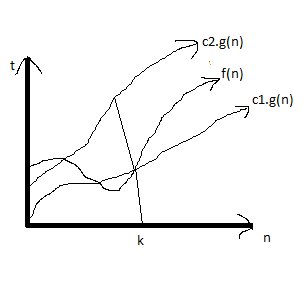### Day 25: Asymptotic Notations

Hello Dear Students,
Hope you all are doing good.

Aaj hum bahut important topic cover karenge which is Asymptotic Notations,

Let's get started...

ASYMPTOTIC NOTATIONS - Asymptotic notations ko algorithms ki complexity define karne ke liye use kiya jata hai. Suppose, we have 2 algorithms, so to find the best algorithm, means jis algorithm ki complexity less hogi wo algorithm best hoga, so ye dekhne ke liye hum asymptotic notations ko use karte hain. Asymptotic notations are as follows-

1. Big Oh (O) Notation - Big Oh notation basically worst case time complexity ko describe karne ke liye use kiya jata hai. Big Oh notation mein upper bound ko consider kiya jata hai. Mathematically,
f(n)= O(g(n)), means
f(n)<= c.g(n), where c=coefficient and g(n) is function which considers the least upper bound.Big Oh Notation (O)

For example, agar hume f(n) diya hoga suppose, 2n2+n, then find out karna hoga Big Oh ko to hum kaise karenge?

• Ab hume f(n) given hai and big oh ke liye f(n)<=c.g(n) hona chahiye. So, hum find out karenge,
• So hum g(n) mein g(n) ki value kya le sakte hain? f(n) se big value honi chahiye, so, f(n) mein n and nhai from which big value is n2 . So, hum g(n) mein n ki value ko n2 lenge.
• c means coefficient, so hum n2 ka coefficient kya le sakte hain ki f(n) se jyada ho? Agar hum 2 lete hain to ye ban gaya 2n2 but ye to f(n) se greater nahi hai, so hum coefficient lenge 3. So, ab ban gaya 3n2 . Which is greater than f(n).
• So, f(n)<=O(g(n))
• 2n2 +n= 3n2
So, Big Oh of 2n2 +n is 3n2 which means algorithm ki time complexity big oh notation mein 3n2 hogi.

2. Big Omega Notation(Ω) - Big Omega notation basically best case time complexity ko describe karne ke liye use kiya jata hai. Big Omega notation mein lower bound ko consider kiya jata hai. Mathematically,
f(n)= Ω (g(n)), means
f(n)>= c.g(n),where c is coefficient and g(n) is function which considers the greatest lower bound.Big Omega Notation (Ω)

Big Omega notation ko understand karne ke liye hum above example hi consider karte hain, So,

For example, agar hume f(n) diya hoga suppose, 2n2+n, then find out karna hoga Big Omega ko to hum kaise karenge?

• f(n) given hai and big omega ke liye f(n)>=c.g(n) hona chahiye. So, hum find out karenge,
• So hum g(n) mein n ki value kya le sakte hain? f(n) mein n and nhai from which small value is n but hum nlenge because greatest lower bound hona chahiye and greatest lower bound is n2 . So g(n) is n2
• c means coefficient, so hum g(n) ka coefficient kya le sakte hain ki f(n) se smaller ho? So, hum 2 lenge coefficient. So, it will be 2n2
• So, f(n)>=Ω(g(n))
• 2n2 +n= 2n2
So, Big Omega notation of 2n2 +n is 2n2 which means algorithm ki time complexity big omega(Ω) notation mein 2n2 hogi.

3. Big Theta Notation(θ) -  Big Theta notation (θ)basically average case time ko describe karne ke liye use kiya jata hai. Big Theta notation mein exact time ko calculate kiya jata hai. Mathematically,
f(n)=θ g(n), means
c1.g(n)<=f(n)<=c2.g(n), where c1 and c2 are coefficients of g(n) and g(n) is function which considers the exact time.Big Theta Notation (θ)

Above example le kar hum big theta ko bhi understand karenge,
• Hume chahiye c1.g(n)<=f(n)<=c2.g(n), in which we have do earlier that g(n) is n2 and less than f(n) is 2n2  and greater than f(n) is 3n2  .
• So, 2n2 <= 2n2 +n <= 3n2
• So, Big Theta of 2n2 +n is 2n2  and 3n2

The above are basic asymptotic notations, we also have little Oh, little Omega and little Theta, but wo itne useful nahi hain so hum ye basic 2 asymptotic notations ko hi consider karenge.
1. Little Oh - Jaise Big Oh mein f(n) <= c.g(n) hota hai, little Oh mein f(n) < c.g(n) hota hai, only equal to nahi nota.
2. Little Omega - Jaise Big Omega mein f(n) >= c.g(n) hota hai, little Omega mein f(n) > c.g(n) hota hai, only equal to nahi hota.
3. Little Theta - Jaise Big Theta mein c1.g(n) <= f(n) <= c2.g(n) hota hai, little theta mein c1.g(n) < f(n) < c2.g(n) hota hai, equal to nahi hota.

COMPLEXITIES
 Algorithms Complexity Linear Search O(n) Binary Search O(log2n) Bubble Sort O(n2 ) Insertion Sort O(n2 ) Selection Sort O(n2 ) Quick Sort O(n2 ) Heap Sort O(n log2n) Merge Sort O(n log2n)

Best of Luck Students,
Do visit our website regularly for more content and for daily tests.

Regards,
UGC NET EXPERTS